Next: Motion in one-dimensional potential Up: Newtonian mechanics Previous: Newton's third law of

# Non-isolated systems

Up to now, we have only considered isolated dynamical systems, in which all of the forces acting on the system originate from within the system itself. Let us now generalize our approach to deal with non-isolated dynamical systems, in which some of the forces originate outside the system. Consider a system of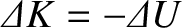mutually interacting point objects. Let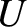and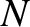be the mass and position vector of the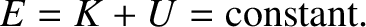th object, respectively. Suppose that theth object is subject to two forces: first, an internal force that originates from the other objects in the system; and, second, an external force that originates outside the system. In other words, let the force acting on theth object take the form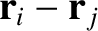(2.38)

where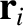is the internal force exerted by object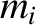on object, and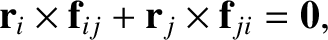the external force acting on object.

The equation of motion of theth object is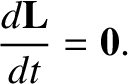(2.39)

Summing over all objects, we obtain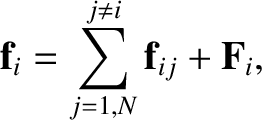(2.40)

which reduces to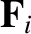(2.41)

where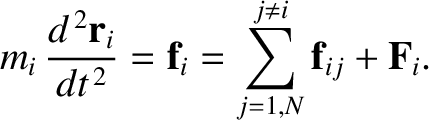(2.42)

is the net external force acting on the system. Here, the sum over the internal forces has cancelled out in pairs as a result of Newton's third law of motion. (See Section 2.5.) We conclude that if there is a net external force acting on the system then the total linear momentum evolves in time according to Equation (2.41) but is completely unaffected by any internal forces. The fact that Equation (2.41) is similar in form to Equation (2.13) suggests that the center of mass of a system consisting of many point objects has analogous dynamics to a single point object, whose mass is the total system mass, moving under the action of the net external force.

Taking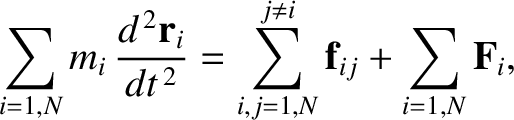Equation (2.39), and summing over all objects, we obtain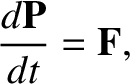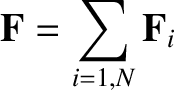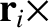(2.43)

where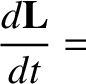(2.44)

is the net external torque (about the origin) acting on the system. Here, the sum over the internal torques has cancelled out in pairs, assuming that the internal forces are central in nature. (See Section 2.5.) We conclude that if there is a net external torque acting on the system then the total angular momentum evolves in time according to the simple equation (2.43) but is completely unaffected by any internal torques.Next: Motion in one-dimensional potential Up: Newtonian mechanics Previous: Newton's third law of
Richard Fitzpatrick 2016-03-31